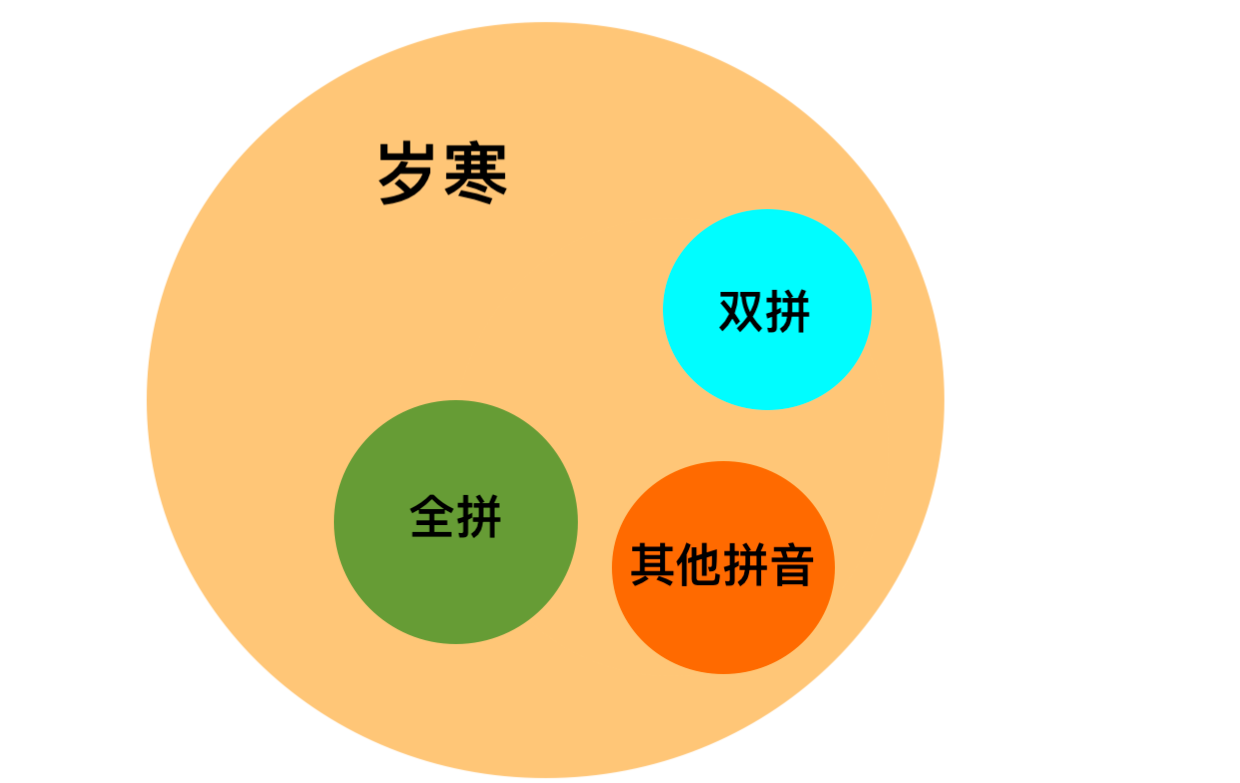### 前言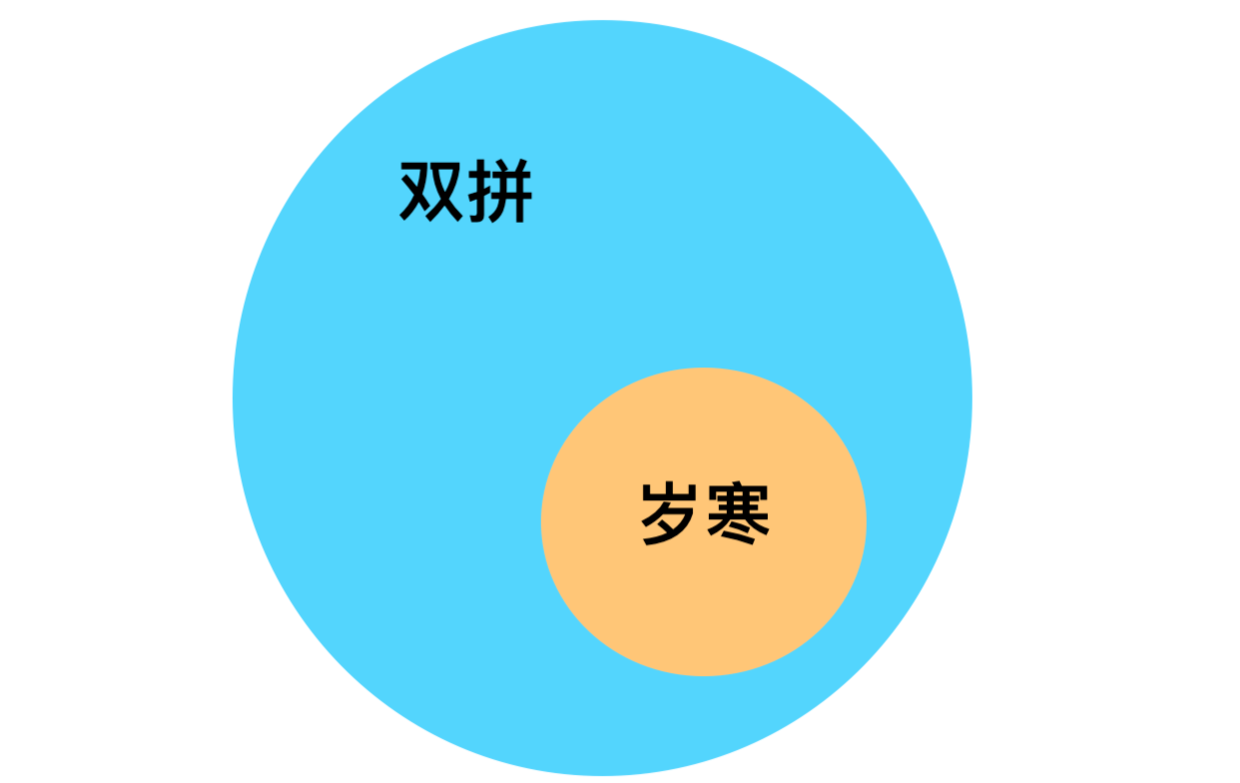### 带条件的前置路径

##### 前置路径表示法##### 条件的组成与要求

1. 任一声母b p m f d t n l ɡ k h j q x zh ch sh r z c s y w和零声母(用数字0表示，关于零声母下文会详细讲解) **解释：**当条件声明为声母时，表示当前输入位置上存在且仅存在所声明的声母时，将指定路径接受为指定的声母或韵母，并输入到当前的输入位置上；示例如：
s:a=ang
//该式表示当当前输入位置为s时，点击a，
//在当前输入位置上输入ang，当前输入位置将变为sang；


（该条件的解释在拼音替入时存在例外，在优先级一节中介绍）

1. 任一韵母或任一韵母的前缀；韵母的数量有点多，这里就不一一列举了。那什么是韵母的前缀呢？韵母的前缀就是由合法韵母从左到右若干个字母组成的字符串，即便不是合法的韵母也可以，如：iong的前缀：io、ion; **解释：**当条件声明为韵母或韵母的前缀时，表示当前输入位置上存在且仅存在所声明的韵母或韵母的前缀时，将指定路径接受为指定的声母或韵母，并输入到当前的输入位置上；示例如：
a:n=an
//该式表示当当前输入位置为a时，点击n，
//在当前输入位置上输入an，当前输入位置将变为an；

1. 下划线+任一韵母或下划线+任一韵母的前缀，以an为例，则形如_an；这里下划线表示韵母的左侧有声母(这里的声母包括零声母)； **解释：**当条件声明为下划线+任一韵母或下划线+任一韵母的前缀时，表示当前输入位置上存在所声明的韵母或韵母的前缀，且存在任一声母，如所指定的输入为声母时，则将指定路径接受为该声母，并输入到当前的输入位置上；如所指定的输入为韵母且该韵母能与已存在的声母匹配时，则将指定路径接受为该韵母，并输入到当前的输入位置上；示例如：
_a:n=an
//该式表示当当前输入位置为形如xa的输入时,
//其中x表示任一声母，比如sa，点击n，
//由于an能与s匹配，因此在当前输入位置上输入an，
//当前输入位置将变为san；


##### 语法糖
• 条件的简写 为了便于描述多个条件下的同一路径的同一输入，允许在一条表达式中，用/分隔多个条件，如：
s/c/z/sh/ch/zh:a=ang
//该式表示当当前输入位置为s、c、z、sh、ch或zh时，点击a，
//在当前输入位置上输入ang；

• 反选声母的写法 当声母条件较多时，为了缩短条件列表，允许在需要排除的声母条件最前面添加~，表示选择所列出来的声母之外的其他所有声母作为条件，如：
~s/c/z/sh/ch/zh:a=ang
//该式表示当当前输入位置为s、c、z、sh、ch或zh之外的其他声母时，点击a，
//在当前输入位置上输入ang；


~:a=ang
//该式表示当当前输入位置存在声母时，点击a，
//在当前输入位置上输入ang；


### 双拼方案实践

##### 自然码方案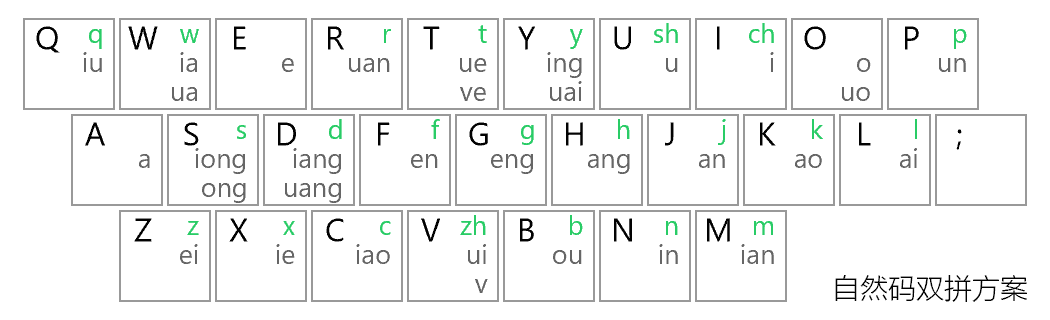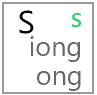##### 自然码中的特殊情况

i=ch
u=sh
v=zh


1. 单字母韵母a，o，e重复按两次。如：a → aa，等。
2. 双字母韵母an，en，ou等不变，直接输入。
3. 三字母韵母ang，eng，重复第一个字母。如：ang → ah （双拼 h = ang）

1. 单字母韵母a，o，e重复按两次。如：a → aa，等。 对于这种情况，我们可以这么写：
a:a=a
o:o=o
e:e=e

1. 双字母韵母an，en，ou等不变，直接输入。 对于这种情况，我们可以这么写：
a:n=an
e:r=er
e:n=en
o:u=ou
a:o=ao
a:i=ai
e:i=ei

1. 三字母韵母ang，eng，重复第一个字母。如：ang → ah （双拼 h = ang）
a:h=ang
e:g=eng


~0:u=u
sh/zh/g/h/k:w=ua
~b/f/j/l/n/m/p/q/w/x/y/0:v=ui
j/l/n/q/x/y:t=ue
~b/f/j/m/p/q/w/x/y/0:o=uo
~b/f/n/m/p/w/0:p=un
sh/ch/zh/g/h/k:y=uai
~b/f/m/p/w/0:r=uan
sh/ch/zh/g/h/k:d=uang
l/n:v=v
~f/g/h/k/w/0:i=i
d/j/l/q/x:w=ia
b/d/j/l/n/m/p/q/t/x:x=ie
b/j/l/n/m/p/q/x/y:n=in
d/j/l/n/m/q/x:q=iu
b/d/j/l/n/m/p/q/t/x/y:y=ing
b/d/j/l/n/m/p/q/t/x:m=ian
b/d/j/l/n/m/p/q/t/x:c=iao
j/l/n/q/x:d=iang
j/q/x:s=iong
~j/q/r/x:a=a
~f/j/q/r/x/y:l=ai
~j/q/x:j=an
~f/j/q/w/x:k=ao
~j/q/x:h=ang
~b/f/j/p/q/w/x:e=e
~ch/zh/c/j/q/r/s/t/x/y:z=ei
~d/j/l/q/t/x/y:f=en
~j/q/x/y/0:g=eng
b/f/m/p/w/y/0:o=o
~b/j/q/w/x:b=ou
~sh/b/f/j/m/p/q/w/x/0:s=ong
i=ch
u=sh
v=zh
a:a=a
o:o=o
e:e=e
a:n=an
e:r=er
e:n=en
o:u=ou
a:o=ao
a:i=ai
e:i=ei
a:h=ang
e:g=eng


##### 针对拼音替入的优化

b4=b
c4=c
d4=d
f4=f
g4=g
h4=h
j4=j
k4=k
l4=l
n4=n
m4=m
p4=p
q4=q
r4=r
s4=s
t4=t
w4=w
x4=x
y4=y
z4=z
04=0
i4/i44=ch
u4/u44=sh
v4/v44=zh


### 给你你想要的真香

##### 岁寒对qwerty布局的改进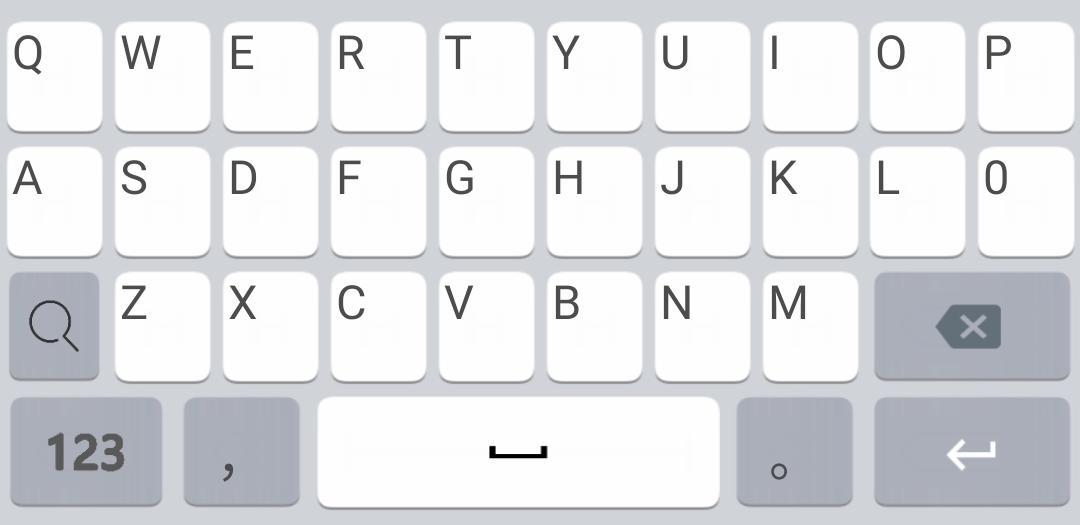##### 助记功能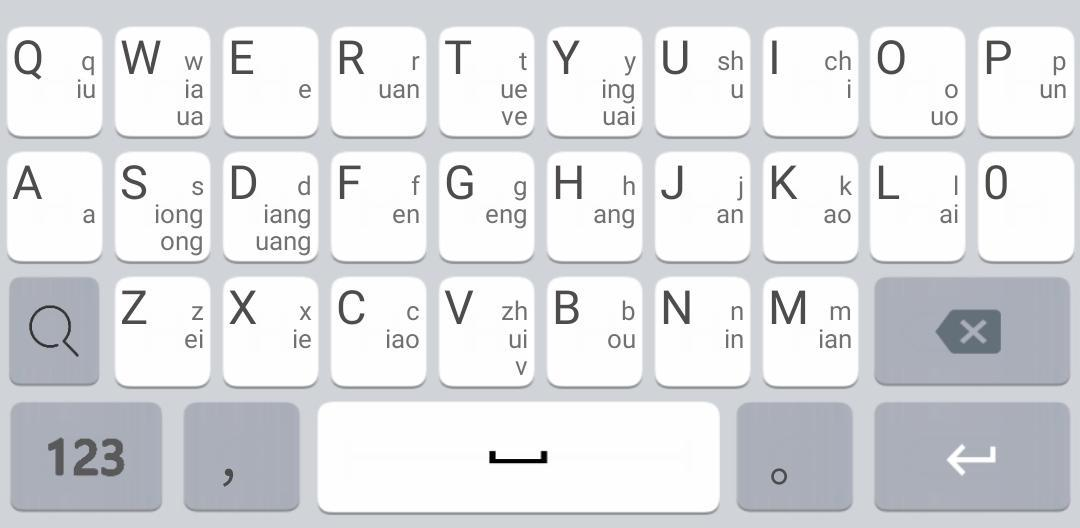### 全拼实践

1. 表示zh/ch/sh：
z:h=zh
c:h=ch
s:h=sh

1. 表示长韵母，以uang为例：
u/_u:a=ua
ua/_ua:n=uan
uan/_uan:g=uang


i/_i:o=io
io/_io:n=ion
ion/_ion:g=iong


_i:o=io
_io:n=ion
_ion:g=iong


z:h=zh
c:h=ch
s:h=sh
a/_a:n=an
an/_an:g=ang
a/_a:i=ai
a/_a:o=ao
o/_o:n=on
on/_on:g=ong
o/_o:u=ou
e/_e:n=en
en/_en:g=eng
e/_e:i=ei
e/_e:r=er
i/_i:n=in
in/_in:g=ing
i/_i:a=ia
ia/_ia:o=iao
ia/_ia:n=ian
ian/_ian:g=iang
i/_i:e=ie
i/_i:o=io
io/_io:n=ion
ion/_ion:g=iong
i/_i:u=iu
u/_u:n=un
u/_u:a=ua
ua/_ua:n=uan
ua/_ua:i=uai
uan/_uan:g=uang
u/_u:o=uo
u/_u:e=ue
u/_u:i=ui
v/_v:e=ue
b4=b
c4=c
d4=d
f4=f
g4=g
h4=h
j4=j
k4=k
l4=l
n4=n
m4=m
p4=p
q4=q
r4=r
s4=s
t4=t
w4=w
x4=x
y4=y
z4=z
04=0
i4/i44=ch
u4/u44=sh
v4/v44=zh


### 写在最后